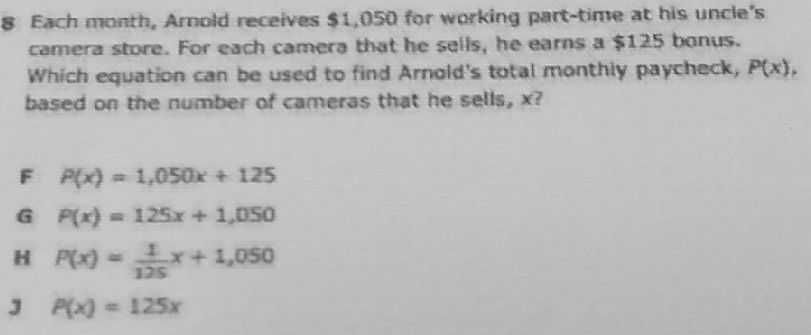### ¿Todavía tienes preguntas de matemáticas?

Pregunte a nuestros tutores expertos
Algebra
Pregunta$$8$$ Each month, Amold receives $$\ 1,050$$ for working part-time at his uncle's camera store. For each camera that he sells, he earns a $$\ 125$$ bonus. Which equation can be used to find Arnold's total monthiy paycheck, $$P ( x )$$ . based on the number of cameras that he selis, $$x ?$$ F $$P ( x ) = 1,050 x + 125$$

$$F ( x ) = 125 x + 1,050$$

$$P ( x ) = \frac { 1 } { 125 } x + 1,050$$

$$3 ( x ) = 125 x$$